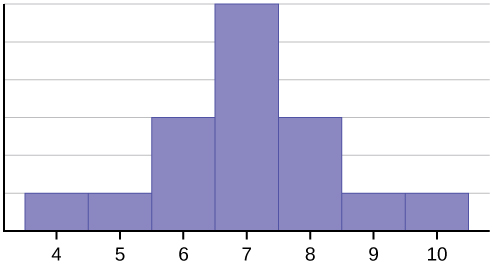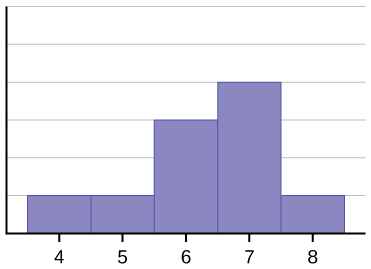# 2.7: Skewness and the Mean, Median, and Mode

$$\newcommand{\vecs}{\overset { \rightharpoonup} {\mathbf{#1}} }$$ $$\newcommand{\vecd}{\overset{-\!-\!\rightharpoonup}{\vphantom{a}\smash {#1}}}$$$$\newcommand{\id}{\mathrm{id}}$$ $$\newcommand{\Span}{\mathrm{span}}$$ $$\newcommand{\kernel}{\mathrm{null}\,}$$ $$\newcommand{\range}{\mathrm{range}\,}$$ $$\newcommand{\RealPart}{\mathrm{Re}}$$ $$\newcommand{\ImaginaryPart}{\mathrm{Im}}$$ $$\newcommand{\Argument}{\mathrm{Arg}}$$ $$\newcommand{\norm}{\| #1 \|}$$ $$\newcommand{\inner}{\langle #1, #2 \rangle}$$ $$\newcommand{\Span}{\mathrm{span}}$$ $$\newcommand{\id}{\mathrm{id}}$$ $$\newcommand{\Span}{\mathrm{span}}$$ $$\newcommand{\kernel}{\mathrm{null}\,}$$ $$\newcommand{\range}{\mathrm{range}\,}$$ $$\newcommand{\RealPart}{\mathrm{Re}}$$ $$\newcommand{\ImaginaryPart}{\mathrm{Im}}$$ $$\newcommand{\Argument}{\mathrm{Arg}}$$ $$\newcommand{\norm}{\| #1 \|}$$ $$\newcommand{\inner}{\langle #1, #2 \rangle}$$ $$\newcommand{\Span}{\mathrm{span}}$$$$\newcommand{\AA}{\unicode[.8,0]{x212B}}$$

Consider the following data set.
4; 5; 6; 6; 6; 7; 7; 7; 7; 7; 7; 8; 8; 8; 9; 10

This data set can be represented by following histogram. Each interval has width one, and each value is located in the middle of an interval.Figure 2.11

The histogram displays a symmetrical distribution of data. A distribution is symmetrical if a vertical line can be drawn at some point in the histogram such that the shape to the left and the right of the vertical line are mirror images of each other. The mean, the median, and the mode are each seven for these data. In a perfectly symmetrical distribution, the mean and the median are the same. This example has one mode (unimodal), and the mode is the same as the mean and median. In a symmetrical distribution that has two modes (bimodal), the two modes would be different from the mean and median.

The histogram for the data: 4; 5; 6; 6; 6; 7; 7; 7; 7; 8 is not symmetrical. The right-hand side seems "chopped off" compared to the left side. A distribution of this type is called skewed to the left because it is pulled out to the left. We can formally measure the skewness of a distribution just as we can mathematically measure the center weight of the data or its general "speadness". The mathematical formula for skewness is:

$a_{3}=\sum \frac{\left(x_{t}-\overline{x}\right)^{3}}{n s^{3}}.\nonumber$

The greater the deviation from zero indicates a greater degree of skewness. If the skewness is negative then the distribution is skewed left as in Figure $$\PageIndex{13}$$.Figure 2.12

The mean is 6.3, the median is 6.5, and the mode is seven. Notice that the mean is less than the median, and they are both less than the mode. The mean and the median both reflect the skewing, but the mean reflects it more so.

The histogram for the data: 6; 7; 7; 7; 7; 8; 8; 8; 9; 10, is also not symmetrical. It is skewed to the right.Figure 2.13

The mean is 7.7, the median is 7.5, and the mode is seven. Of the three statistics, the mean is the largest, while the mode is the smallest. Again, the mean reflects the skewing the most.

To summarize, generally if the distribution of data is skewed to the left, the mean is less than the median, which is often less than the mode. If the distribution of data is skewed to the right, the mode is often less than the median, which is less than the mean.

As with the mean, median and mode, and as we will see shortly, the variance, there are mathematical formulas that give us precise measures of these characteristics of the distribution of the data. Again looking at the formula for skewness we see that this is a relationship between the mean of the data and the individual observations cubed.

$a_{3}=\sum \frac{\left(x_{i}-\overline{x}\right)^{3}}{n s^{3}}\nonumber$

where ss is the sample standard deviation of the data, $$\mathrm{X}_{i}$$, and $$\overline{x}$$ is the arithmetic mean and $$n$$ is the sample size.

Formally the arithmetic mean is known as the first moment of the distribution. The second moment we will see is the variance, and skewness is the third moment. The variance measures the squared differences of the data from the mean and skewness measures the cubed differences of the data from the mean. While a variance can never be a negative number, the measure of skewness can and this is how we determine if the data are skewed right of left. The skewness for a normal distribution is zero, and any symmetric data should have skewness near zero. Negative values for the skewness indicate data that are skewed left and positive values for the skewness indicate data that are skewed right. By skewed left, we mean that the left tail is long relative to the right tail. Similarly, skewed right means that the right tail is long relative to the left tail. The skewness characterizes the degree of asymmetry of a distribution around its mean. While the mean and standard deviation are dimensionalquantities (this is why we will take the square root of the variance ) that is, have the same units as the measured quantities $$\mathrm{X}_{i}$$, the skewness is conventionally defined in such a way as to make it nondimensional. It is a pure number that characterizes only the shape of the distribution. A positive value of skewness signifies a distribution with an asymmetric tail extending out towards more positive $$X$$ and a negative value signifies a distribution whose tail extends out towards more negative $$X$$. A zero measure of skewness will indicate a symmetrical distribution.

Skewness and symmetry become important when we discuss probability distributions in later chapters.

This page titled 2.7: Skewness and the Mean, Median, and Mode is shared under a CC BY license and was authored, remixed, and/or curated by .